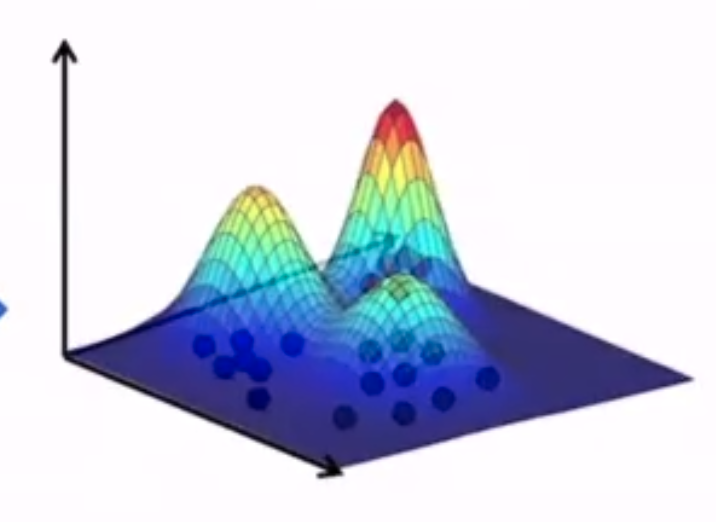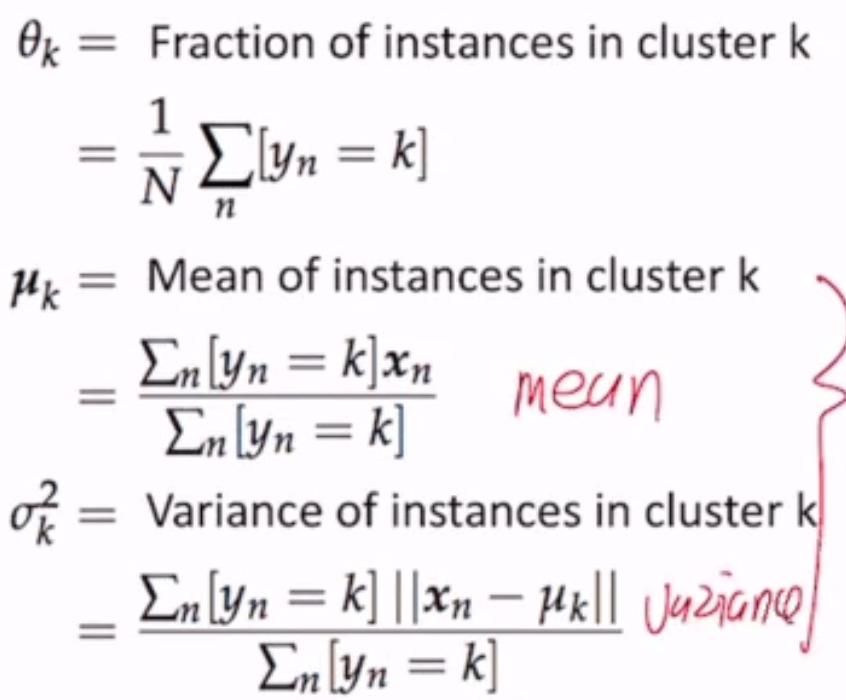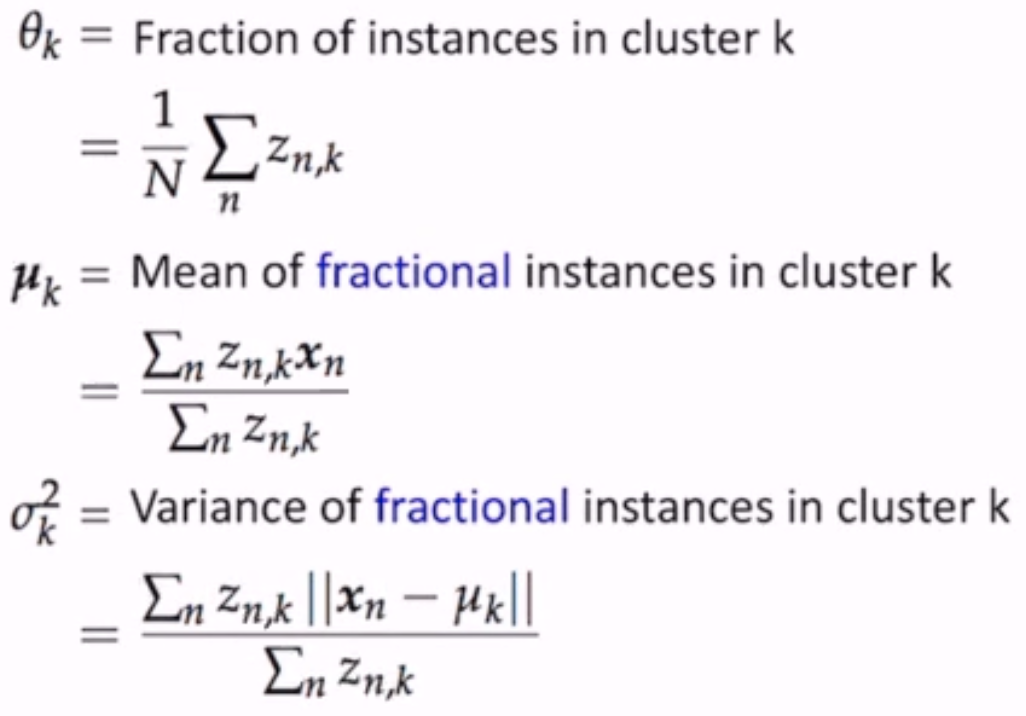# Unsupervised Learning¶

Getting supervised data is expensive and time-consuming; let’s make sense of the data w/o labels

Today: K-means clustering

• Algorithm
• Convergence
• Initialization
• Hyperparameter selection
• how many classes?

In some cases, different clustering algorithms can output different clusters (e.g. multicolored squares/circles - split on shape or color?)

## Algorithm¶

In a clustering problem, each instance is just a data vector $$\mathbf{x}$$ without a label.

Let’s use k-means clustering.

Pseudocode:

assign cluster centers randomly
while centers are changing:
assign each example to the closest center (least square distance)
compute the new centers according to the assignments


But note that there can be many stop conditions - a certain number of iterations, when the centers stop changing, or some other rule

  1 2 3 4 5 6 7 8 9 10 11 12 13 def k_means(D, k): for k = 1 to K: # random init mu_k = some random location while mus changing: for n = 1 to N: # assign to closest center z_n = argmin_k ||u_k - x_n|| for k = 1 to K: # compute new centers X_k = {x_n | z_n = k} mu_k = Mean(X_k) return z 

## Convergence¶

K-means is guaranteed to converge - i.e. there is some loss function, and it stops changing after some time.

So what does it optimize? The sum of the squared distance of points from their cluster means!

$L(z,\mu; D) = \sum_N ||x_n - \mu_{z_n}||^2 = \sum_k \sum_{n:z_n=k} ||x_n - \mu_k||^2$

where $$z$$ is the assignments of each point and $$\mu$$ is the location of each cluster center.

Lemma 1: Given a set of K points, the point m that minimizes the squared distances of all points to m is the mean of the K points.

Theorem: For any dataset and any number of clusters, the K-means algorithm converges in a finite number of iterations.

How to prove:

• focus on where in the algorithm the loss is decreasing
• lines 7, 11
• prove that loss can only decrease at those points
• at line 7, $$||x_n - \mu_b|| \leq ||x_n - \mu_a||$$ where a is old and b is new
• at line 11, by lemma 1, the total sum of the squared distances decreases as the mean is the point that minimizes the squared distances of all points in each cluster
• prove that loss cannot decrease forever - there exists a lower bound reachable in a finite number of steps
• the lower bound is 0, when each training point is the center of a cluster
• $$\mu$$ is always the means of some subset of data, and there are a finite number of values for $$\mu$$

This proves convergence.

But… what does it converge to?

• it’s not guaranteed to converge to the “right answer”
• not even guaranteed to converge to the same answer each time (depends on initialization)

## Initialization¶

How do we find the best answer given that it depends on initialization?

1. run a lot of times and pick the solution with mimimum loss
2. pick certain initialization points

### Furthest-First¶

• pick initial means as far from each other as possible
• pick a random example and set it as the mean of the first cluster
• for 2..K, pick the example furthest away from each picked one

Note: this initialization is sensitive to outliers, but this effect is usually reduced after the first few iterations

Probabilistic Tweak

• pick a random example and set it as the mean of the first cluster
• for 2..K, $$\mu_k$$ is sampled from points in the dataset s.t. the probability of choosing a point is proportional to its distance from the closest other mean
• has better theoretical guarantees

## How to choose K¶

How do we choose K when there are an unknown number of clusters?

• if you pick K with the smallest loss, K=n has loss = 0
• “regularize” K via the BIC/AIC (Bayes Info Criteria/Akaike IC)
• increasing K decreases the first term, but increases the second term

BIC: (where D is the # of training instances)

$\arg \min_K \hat{L}_K + K \log D$

AIC:

$\arg \min_K \hat{L}_K + 2KD$

## Probabilistic Clustering¶

Unobserved Variables

• also called hidden/latent variables
• not missing values; just not observed in the data
• e.g.:
• imaginary quantity meant to provide simplification
• a real world object/phenomena that is difficult or impossible to measure
• a object/phenomena that was not measured (due to faulty sensors)
• can be discrete or continuous

On to the clustering

Let’s assume that each cluster is generated by a Gaussian distribution:The three clusters are defined by $$<\mu_x, \sigma^2_x>$$ for each cluster x.

Additionally, we add a parameter $$\theta = <\theta_R, \theta_G, \theta_B>$$ which is the probability of being assigned to a given cluster.

So now, our objective is to find $$(\theta, <\mu_R, \sigma^2_R>, <\mu_G, \sigma^2_G>, <\mu_B, \sigma^2_B>)$$ - but to find these parameters we need to know the assignments, but the assignments define the parameters!

### Setup¶

Assume that samples are drawn from K Gaussian distributions.

1. If we knew cluster assignments, could we find the parameters?
2. If we knew the parameters, could we find the cluster assignments?

Just as in non-probabilistic k-means, we initialize parameters randomly, then solve 1 and 2 iteratively.

#### Params from Assignments¶

Let the cluster assignment for a point $$x_n$$ be $$y_n$$, and N be the normal distribution.

$\begin{split}P(D|\theta, \mu s, \sigma s) & = P(X, Y | \theta, \mu s, \sigma s) \\ & = \prod_n P(x_n, y_n | \theta, \mu s, \sigma s) \\ & = \prod_n P(y_n | \theta, \mu s, \sigma s) P(x_n | y_n, \theta, \mu s, \sigma s) \\ & = \prod_n P(y_n | \theta) P(x_n| \mu_{y_n}, \sigma_{y_n}) \\ & = \prod_N \theta_{y_n} N(x_n | \mu_{y_n}, \sigma_{y_n})\end{split}$

To find the distributions, we just argmax this equation. But since it’s all products, we can take the log and set the derivatives to 0!

$\log P(D|\theta, \mu s, \sigma s) = \sum_n \log \theta_{y_n} + \sum_n \log N(x_n | \mu_{y_n}, \sigma_{y_n})$#### Assignments from Params¶

Assign a point to the most likely cluster:

$\begin{split}y_n & = \arg \max_k P(y_n = k | x_n) \\ & = \arg \max_k \frac{P(y_n = k, x_n)}{P(x_n)} \\ & = \arg \max_k P(y_n = k, x_n) \\ & = \arg \max_k P(y_n = k) P(x_n | y_n = k) \\ & = \arg \max_k \theta_k N(x_n|\mu_k, \sigma^2_k)\end{split}$

Note though - each point has exactly one cluster assignment. But we live in a probabilistic world - assign the point to every cluster with a probability!

Soft Assignment

$$z_{n,k}$$ is a real number in the range [0..1], $$Z_n$$ is a normalization constant.

$\begin{split}z_{n,k} & = P(y_n = k | x_n) \\ & = \frac{P(y_n = k, x_n)}{P(x_n)} \\ & = \frac{1}{Z_n} P(y_n = k, x_n) \\ & = \frac{1}{Z_n} P(y_n = k) P(x_n | y_n = k) \\ & = \frac{1}{Z_n} \theta_k N(x_n|\mu_k, \sigma^2_k)\end{split}$

and $$\sum_k z_{n,k} = 1$$.

• find the parameters that maximize the likelihood of the dataThis procedure is called Expectation Maximization, where step 1 is the Maximization step and step 2 is the Expectation step. This is the Gaussian Mixture Model, specifically

In conclusion, EM:

1. computes the expected values for the latent values/params (in gaussian, $$\mu, \sigma^2$$)
2. maximizes the expected complete log likelihood of the data to estimate the parameters/latent vars

Note

K-means is a special form of GMM, and GMM is a special form of EM-clustering.